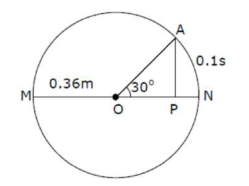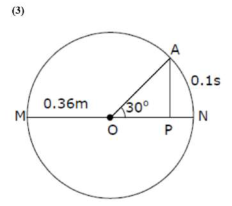# The point A moves with a uniform speed along the circumferenceQuestion:

The point A moves with a uniform speed along the circumference of a circle of radius $0.36 \mathrm{~m}$ and cover $30^{\circ}$ in $0.1 s$. The perpendicular projection 'P' form ' $A^{\prime}$ on the diameter MN represents the simple harmonic motion of 'P'. The restoration force per unit mass when P touches M will be:1. (1) $100 \mathrm{~N}$

2. (2) $50 \mathrm{~N}$

3. (3) $9.87 \mathrm{~N}$

4. (4) $0.49 \mathrm{~N}$

Correct Option: , 3

Solution:The point a covers $30^{\circ}$ in $0.1$ sec.

Means $\frac{\pi}{6} \longrightarrow 0.1 \mathrm{sec}$

$1 \longrightarrow \frac{0.1}{\frac{\pi}{6}}$

$2 \pi=\longrightarrow \frac{0.1 \times 6}{\pi} \times 2 \pi$

$\mathrm{T}=1.2 \mathrm{sec}$

We know that $\omega=\frac{2 \pi}{\mathrm{T}}$

$\omega=\frac{2 \pi}{1.2}$

Restoration force $(F)=m \omega^{2} A$

Then Restoration force per unit mass $\left(\frac{F}{\mathrm{w}}\right)=\omega^{2} \mathrm{~A}$

$\left(\frac{F}{m}\right)=\left(\frac{2 \pi}{1.2}\right)^{2} \times 0.36$

$\cong 9.87 \mathrm{~N}$